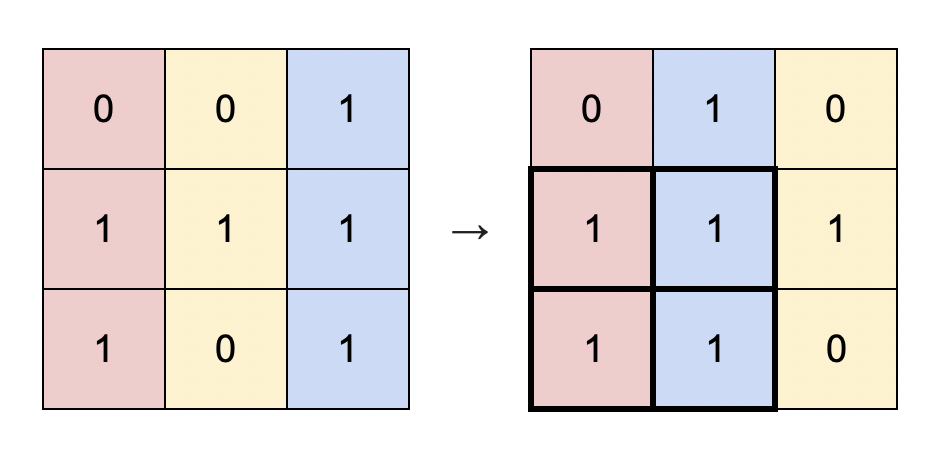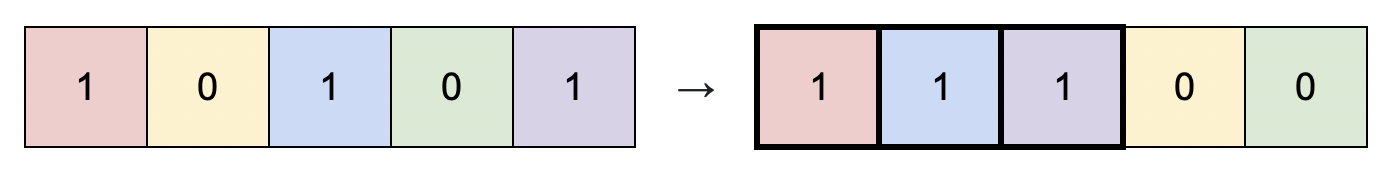# GeetCode Hub

You are given a binary matrix `matrix` of size `m x n`, and you are allowed to rearrange the columns of the `matrix` in any order.

Return the area of the largest submatrix within `matrix` where every element of the submatrix is `1` after reordering the columns optimally.

Example 1:```Input: matrix = [[0,0,1],[1,1,1],[1,0,1]]
Output: 4
Explanation: You can rearrange the columns as shown above.
The largest submatrix of 1s, in bold, has an area of 4.
```

Example 2:```Input: matrix = [[1,0,1,0,1]]
Output: 3
Explanation: You can rearrange the columns as shown above.
The largest submatrix of 1s, in bold, has an area of 3.
```

Example 3:

```Input: matrix = [[1,1,0],[1,0,1]]
Output: 2
Explanation: Notice that you must rearrange entire columns, and there is no way to make a submatrix of 1s larger than an area of 2.```

Example 4:

```Input: matrix = [[0,0],[0,0]]
Output: 0
Explanation: As there are no 1s, no submatrix of 1s can be formed and the area is 0.```

Constraints:

• `m == matrix.length`
• `n == matrix[i].length`
• `1 <= m * n <= 105`
• `matrix[i][j]` is `0` or `1`.

class Solution { public int largestSubmatrix(int[][] matrix) { } }